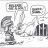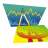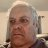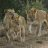# Why won't this strategy plot?##### Well-known member
I've been working on a options strategy and really want to test it so I can get a better understanding on how I can improve it. My goal was to have a buy condition and then sell on the opening of the 4th day. I'm pretty sure I did this a very unorthodox way, it plots arrows, yet It won't work in a strategy. Hopefully someone can help. Does anyone know a simpler way to make the sell condition "sell after 3 days"
Code:
``````input price = close;
input length4 = 13;
input displace = 0;
plot AvgExp = ExpAverage(price[-displace], length4);
input length6 = 48.5;
plot AvgExp2 = ExpAverage(price[-displace], length6);
def c = close;

input length = 14;
input calcLength = 5;
input smoothLength = 3;
input length2 = 30;
input calclength2 = 5;
input smoothlength2 = 2;
input agg = AggregationPeriod.DAY;
input agg2 = AggregationPeriod.THREE_DAYS;

def zeroline = 0;
def o10 = open(period = agg);
def c10 = close(period = agg);
def data = fold i = 0 to length2
with s
do s + (if c10 > GetValue(o10, i)
then 1
else if c10 < GetValue(o10, i)
then - 1
else 0);
def EMA5 = ExpAverage(data, calclength2);
def Main = ExpAverage(EMA5, smoothLength);
def Signal = ExpAverage(Main, smoothLength);

def zero = if IsNaN(c) then Double.NaN else 0;
def ob = if IsNaN(c) then Double.NaN else Round(length * .7);
def ob2 = if IsNaN(c) then Double.NaN else Round(length * .3);
def  ob3 = if IsNaN(c) then Double.NaN else Round(length * .0504);
def ob4 = if IsNaN(c) then Double.NaN else Round(length * -.7);
def ob5 = if IsNaN(c) then Double.NaN else Round(length * -.3);
def ob6 = if IsNaN(c) then Double.NaN else Round(length * -.2);
def ob7 = if IsNaN(c) then Double.NaN else Round(length * .0);
def os = if IsNaN(c) then Double.NaN else -Round(length * .2);
def os2 = if IsNaN(c) then Double.NaN else -Round(length * .6);
def os3 = if IsNaN(c) then Double.NaN else -Round(length * .0);
def os4 = if IsNaN(c) then Double.NaN else -Round(length * -.1);

def o2 = open(period = agg2);
def c2 = close(period = agg2);
def data2 = fold i2 = 0 to length
with s2
do s2 + (if c2 > GetValue(o2, i2)
then 1
else if c2 < GetValue(o2, i2)
then - 1
else 0);

def EMA52 = ExpAverage(data2, calclength2);
def Main2 = ExpAverage(EMA52, smoothLength);
def Signal2 = ExpAverage(Main2, smoothLength);

input ShowTodayOnly = yes;
input ShowEntryExitBands = yes;
input ShowBubbles = yes;
input SellEntry = 3;
input SellExit = 20;

input ATRLength = 50;
input TargetATRMult = 1;
input DisplayLines = yes;
input PriceDigit = 2;

# High

def H1  = Highest(high, SellExit);
def H2  = fold i3 = 1 to SellExit
with ip = 0.0
do if GetValue(high, i3) == H1 or GetValue(high, i3) < ip
then ip
else GetValue(high, i3);
def H3  = fold i1 = 1 to SellExit
with ip1 = 0.0
do if GetValue(high, i1) == H1 or GetValue(high, i1) == H2 or GetValue(high, i1) < ip1
then ip1
else GetValue(high, i1);
def HH  = (H2 + H3) / 2.0;

# Low

def L2  = fold i4 = 1 to BuyExit
with ip2 = 10000000.0
do if GetValue(low, i4) == L1 or GetValue(low, i4) > ip2
then ip2
else GetValue(low, i4);
def L3  = Lowest(if low == L1 or low == L2 then 1000000 else low, BuyExit);
def LL  = (L2 + L2) / 2.0;

def QS = Lowest(low, SellEntry);
plot x = QB;
plot y = ll;
def ATRVal = ATR(length = ATRLength, averageType = AverageType.SIMPLE);
def mATR = Highest(ATRVal, ATRLength);
plot reversethatb = (high - open > 0) and (open > close);

plot optionbuy = (close > QB) and ((Main - 2 > Main) or (main > ob)) and (close < QB) and (close < QB) and (close < QB);

, price =  open[-1], 1, tickcolor = Color.GREEN, arrowcolor = Color.GREEN, name = "BE");

, price = open[-1], 1, tickcolor = Color.RED, arrowcolor = Color.RED, name = "SE");``````#### BenTen

Staff
VIP
You need to add it as a strategy and it will plot your order. Here I have MSFT on the 1Y 1D chart with the code you posted.thanks

R

#### RickAns

##### Member
VIP
Is there an easy way to look at some code and tell whether it is a Study or a Strategy?#### BenTen

Staff
VIP
@RickAns All strategy will have AddOrder in their code.

•RickAnsWhy Some Indicators Won't Work on ThinkorSwim Mobile App Tutorials 11Easycator's VWAP Trading Indicator/Strategy Questions 12Strategy Sell before Buy Questions 7RSI Stochastic Strategy Questions 3